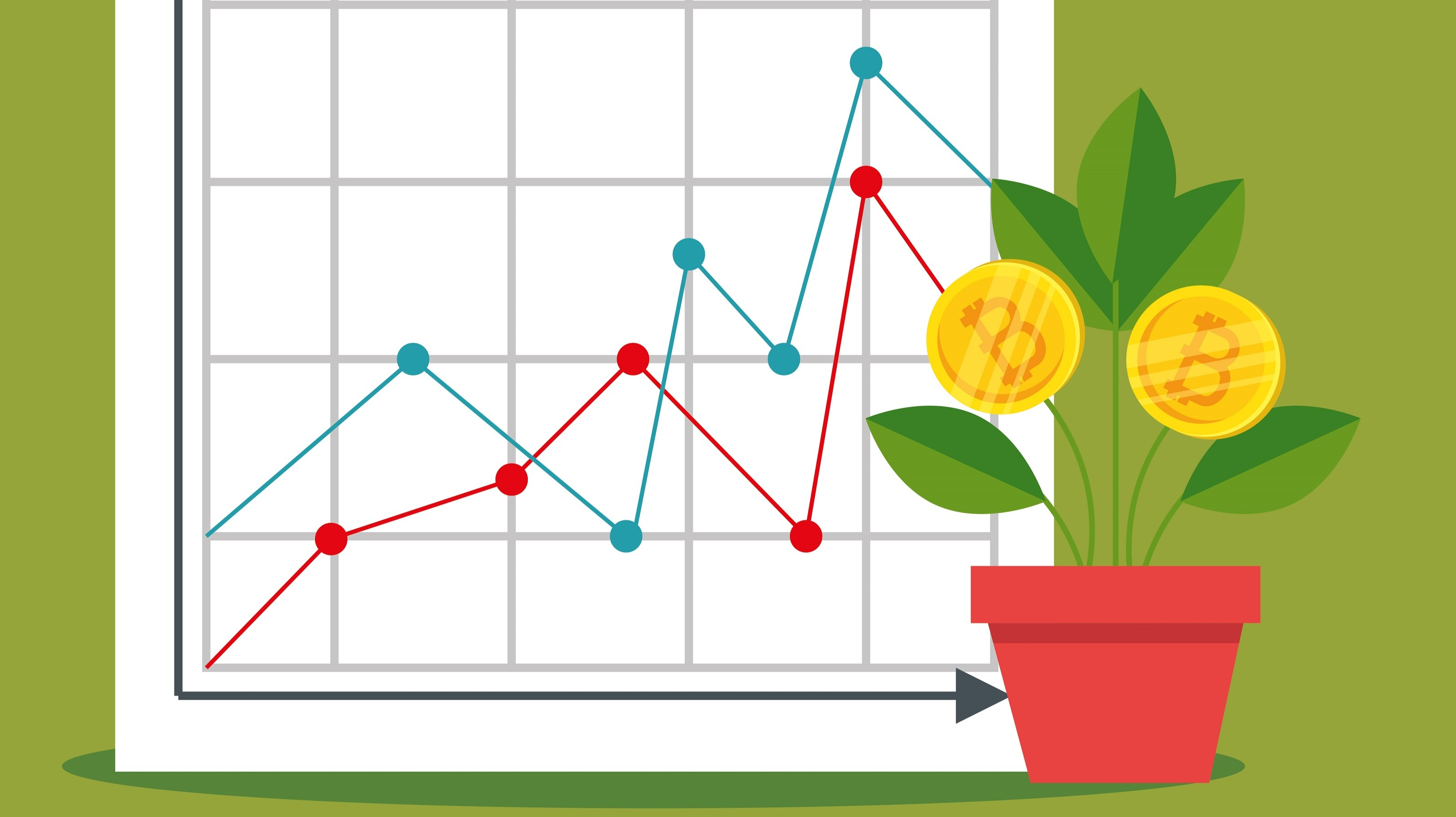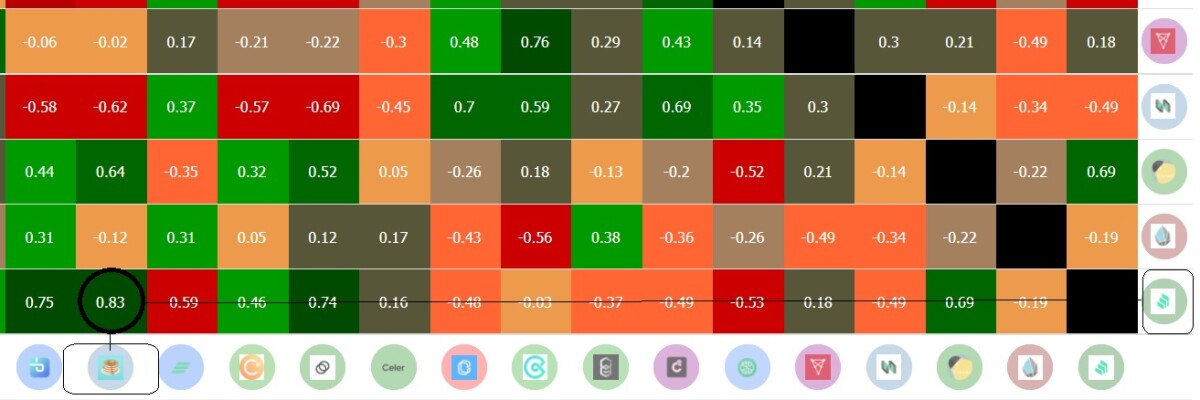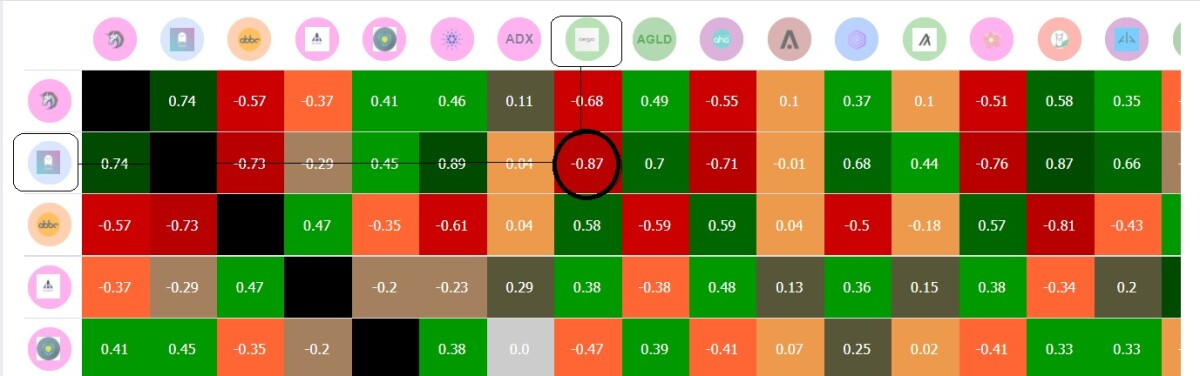# Maximize your Crypto Profit by Using Correlation - A beginner guide

Correlation is the fundamental concept and indispensable measure in statistical analyses which has a broad range of applications in diverse businesses and industries. The aim of this short blog post is to describe how correlation can be used in cryptocurrency investment and trading.

Firstly, I will briefly explain the definition of statistical correlation, then will go on to the application of correlation analysis in cryptocurrency.  Finally, I will introduce an online free tool for correlations of cryptos.

#### Correlation in statistics

Correlation is a statistical measure that expresses the extent to which two variables are linearly related (meaning they change together at a constant rate). It is a common tool for describing simple relationships without making a statement about cause and effect.

Two variables can have positive, negative or no correlation:

• A positive correlation is a relationship between two variables in which they go up and down hand in hand. An example of a positive correlation would be height and weight. Taller people tend to be heavier.
• A negative correlation is a relationship between two variables in which an increase in one variable is associated with a decrease in the other. An example of a negative correlation would be bicycle traffic and rain. Heavier rain leads to less use of bicycles.

#### Correlation in Cryptocurrency

When two crypto prices are associated in a clear pattern, for example, when high prices on one consistency go with high prices on the other, and lows on one go with lows on the other, the two cryptos are correlated. This can happen, for instance, when the two cryptos are nested in the same ecosystem. Statistically speaking, these two variables are positively correlated. Two cryptos can have a negative correlation as well. Meaning that a high price on one goes with a low price on the other.

Finding this pattern in cryptos can be useful in two ways:

##### Risk management in portfolio

Risk management is an important aspect of investment. To minimize the risk and reduce the portfolio volatility, one strategy would be diversifying the portfolio or holding diversified coins or tokens. Therefore, one crucial question in taking this strategy is how can we have a diversified portfolio. In other words, what pool of cryptos is broadly diverse. Correlation analysis can answer this question. To have a diversified portfolio you should avoid coins that are highly (positively) correlated.

Correlation analysis can be useful in trading as well. Assume that coin A has a high (positive) correlation with B and a high (negative) correlation with coin C. When the price of coin A goes up, you can expect that the price of coin B follows the same, and the price of coin C falls down. Therefore, if you are left behind buying coin A, you can buy coin B (or any other coins that are highly (positively) correlated with A) and consider selling coin C (or any other coins that are highly (negatively) correlated with A).

#### Correlation Table

There is plenty of websites that provide you with a correlation table or chart; such as CryptoWatch and CoinMetrics. However, I am going to introduce a website that I have recently encountered and that is easy to use for beginners: Macroaxis.

In MacroAxis (https://www.macroaxis.com/) you should go Crypto Center and then select Correlations.

Correlation measures are always from -1 to +1 (from highly negative correlation to highly positive correlation). In correlation tables you can find  correlations between two coins or tokens. Each cell in the table represents the correlation between correspondent raw and column.

For instance, in the below table we can see Compound and PancakeSwap are highly correlated (+.83).And from the table below we can see AAVE (a lending and borrowing platform) and AERGO are highly negatively correlated (-0.87).#### Conclusion

Correlation is a method to measure the relationship between two variables. This measure is commonly used in many areas and fields. In cryptocurrency trading and investment can be easily used in two ways. As a holder, you can manage the diversity of your portfolio thanks to correlation analysis, and as a trader, you can predict the movement of coins' price by having considered the correlation between different coins. To find correlations, you can use online free websites that provide correlation tables such as macroaxis.com, coinmetrics.io, and cryptowat.ch.

##### Suggested passive income:
• Stake, Add liquidity and earn: Sign-up CakeDefi (Earn 15\$ sign-up bonus + \$5 learn and earn in DFI) - (Referral link)
• Share your internet traffic and earn: HoneyGainSociology of Blockchain

This blog contributes to understanding blockchain society interaction.Send a \$0.01 microtip in crypto to the author, and earn yourself as you read!

20% to author / 80% to me.
We pay the tips from our rewards pool.